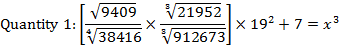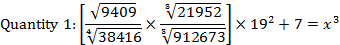# Quadratic Equation New Pattern Questions

Directions.1-5) Solve the two given quantities and find out the relationship between them.

Q.1) Quantity 1: Area of square having its perimeter is 2 times of circumference of circle having 21mtrs as its radius.

Quantity 2: Area of a rectangle whose length is 150% of its breadth and which perimeter is 270mtrs.

a) Quantity 1 > Quantity 2

b) Quantity 1 < Quantity 2

c) Quantity 1 ≥ Quantity 2

d) Quantity 1 ≤ Quantity 2

e) Quantity 1 = Quantity 2 or Relationship can’t be established.

b

Quantity 1:

Circumference of square = 2πr

= 2×22/7×21

= 132mtrs

Perimeter of square = 2 times of the circumference of circle = 132 × 2 = 264mtrs

Side of Square a =264/4= 66mtrs

Area of square = a2= 662  = 4356sq.mtrs

Quantity 2:

Perimeter = 2(L+ B)= 270mtrs

L+B = 135 mtrs

L =150% of B

Then L and B = 81, 54 respectively

Area of the rectangle = L×B = 81 × 54 = 4374sq.mtrs

So, Quantity 1 <Quantity 2.

Q.2) Quantity 1 = 75x7÷(y3z2)

Quantity 2 = (23y4x3)÷z5

X = 1 ; Y = 2 ; Z =3

a) Quantity 1 > Quantity 2

b) Quantity 1 < Quantity 2

c) Quantity 1 ≥ Quantity 2

d) Quantity 1 ≤ Quantity 2

e) Quantity 1 = Quantity 2 or Relationship can’t be established.

Quantity 1:

= 75x7÷(y3 z2 )

=75(17 )/(23 32 )

=75/(8×9)

=75/72= 1.04

Quantity 2:

=(23 ×24×1)3/35

=  (23 ×16)/243

=368/243

= 1.51

So Quantity 1 <Quantity 2.

Q.3)

Quantity 1: Perimeter of a Square having its side is equal to hypotenuse of a right-angled triangle having its sides 8mtrs and 6mtrs respectively.

Quantity 2: Perimeter of a Rhombus having its diagonals d1 and d2 are 12mtr and 16mtr respectively.

a) Quantity 1 > Quantity 2

b) Quantity 1 < Quantity 2

c) Quantity 1 ≥ Quantity 2

d) Quantity 1 ≤ Quantity 2

e) Quantity 1 = Quantity 2 or Relationship can’t be established.

Quantity 1:

Hypotenuse of a triangle = √(a2+b2)

=  √(82+62 )

=  √(64+36)

=  √100

= 10 mtrs

And Perimeter of the square = 4a = 4 × 10 = 40mtrs.

Quantity 2:

Diagonals of rhombus intersect at 90°angle.

The diagonals and the sides of the rhombus form right triangle.

One side of the right triangle is 16/2= 8mtrs

Other side of the right triangle is 12/2= 6mtrs

Then the third side of the triangle = √(a2+b2 )

=  √(82+62 )

= 10mtrs

And Perimeter of the rhombus = 4a = 4 × 10 = 40mtrs.

So Quantity 1 is equal to Quantity 2.

Q.4)

Quantity 1: The value of “a” from 8a2+6a+10=15

Quantity 2: The value of “b” from 12b2+9b = 7.5

a) Quantity 1 > Quantity 2

b) Quantity 1 < Quantity 2

c) Quantity 1 ≥ Quantity 2

d) Quantity 1 ≤ Quantity 2

e) Quantity 1 = Quantity 2 or Relationship can’t be established.

e

Quantity 1:

= 8a2+ 6a + 10 = 15

= 8a2+ 6a– 5 = 0

= 8a2– 4a + 10a– 5 = 0

= 4a (2a-1)+ 5(2a-1)= 0

i) 4a + 5 = 0, ii) 2a- 1= 0

i) 4a + 5 = 0

4a = -5

A = -5/4

A = -1.25

ii) 2a– 1 = 0

2a = 1

A = ½ = 0.5

Then the values of A = -1.25, 0.5

Quantity 2:

12b2+ 9b– 7.5 = 0

12b2– 6b + 15b– 7.5 = 0

6b (2b– 1)+ 7.5 (2b– 1)= 0

i) (2b– 1), ii) (6b + 7.5) = 0

i) (2b– 1)

2b = 1

B =1/2= 0.5

ii) (6b + 7.5)

6b = -7.5

B = -7.5/6

B = -1.25

Then the values of B = -1.25 and 0.5

So the Relationship can’t be established.

Q.5)

Quantity 1: The value of X from 1/(x-3) – 1/(x+5)=1/6

Quantity 2: The value of X from x3+ y3+ z3– 3xyz =0, if the value of y = 6 and z = -10.

a) Quantity 1 > Quantity 2

b) Quantity 1 < Quantity 2

c) Quantity 1 ≥ Quantity 2

d) Quantity 1 ≤ Quantity 2

e) Quantity 1 = Quantity 2 or Relationship can’t be established.

Quantity 1:

((x+5)–(x+3))/(x-3)(x+5) =1/6

8/(x2+2x-15)  =1/6

48 = x2+ 2x – 15

48 + 15 = x2+2x

63 = x2+2x

(x+9)(x-7)= 0

Then x = 7 and -9

Quantity 2:

x3+ y3+ z3– 3xyz =0

x3+ y3+ z3– 3xyz = 0=(x+y+z)

x+6-10 = 0

x – 4 = 0

Then x = 4

So the Relationship can’t be established.

Directions.6-10) In the following question two quantities are given Quantity 1 and quantity 2 By solving those quantities give corresponding answer.

Q.6)a) Quantity 1 ≥ Quantity 2

b) Quantity 1 = Quantity 2 (or) No relation

c) Quantity 1 > Quantity 2

d) Quantity 1 ≤ Quantity 2

e) Quantity 1 < Quantity 2

a) Quantity 1 ≥ Quantity 2

Q.7)Quantity 1  :The arthmetic mean of 1,2,4,8,…250

Quantity 2 :The geometric mean of 1,4,16,…425

a) Quantity 1 ≥ Quantity 2

b) Quantity 1 = Quantity 2 (or) No relation

c) Quantity 1 > Quantity 2

d) Quantity 1 ≤ Quantity 2

e) Quantity 1 < Quantity 2

b) Quantity 1 = Quantity 2 (or) No relation

Q.8)

Quantity 1:A wooden wedge is in the shape of a sector of circle of radius 15cm with angle 48° and constant thickness of 6cm. Find the volume of the wood used in making the wedge

Quantity 2:Find the volume of a hemisphere whose radius is 7cm.

a) Quantity 1 ≥ Quantity 2

b) Quantity 1 = Quantity 2 (or) No relation

c) Quantity 1 > Quantity 2

d) Quantity 1 ≤ Quantity 2

e) Quantity 1 < Quantity 2

e) Quantity 1 < Quantity 2

Q.9)

Quantity 1: In a company 30% of the workers were females and their annual average income is 720 and the remaining 70% workers were males and their annual salary is 360. What is the average annual income of all the workers.

Quantity 2: The average income of a family having 3 male and two female members is 6000.when one male member of the family whose salary  is 800 has left and is replaced by a women the average of the family gets reduced by 20.then what is new women’s salary

a) Quantity 1 ≥ Quantity 2

b) Quantity 1 = Quantity 2 (or) No relation

c) Quantity 1 > Quantity 2

d) Quantity 1 ≤ Quantity 2

e) Quantity 1 < Quantity 2

e) Quantity 1 < Quantity 2

Q.10)

Quantity 1:A and B can do a work in 12 days and 15 days respectively. they began to work together after 3 days B had left the remaining work was completed by A with help of c in 3 days. C alone can do the work in

Quantity 2: Two persons P1 and P2 together can complete a work in 24 days and person P3 can do the work in 36 days .If all three work together the work will be completed in

a) Quantity 1 ≥ Quantity 2

b) Quantity 1 = Quantity 2 (or) No relation

c) Quantity 1 > Quantity 2

d) Quantity 1 ≤ Quantity 2

e) Quantity 1 < Quantity 2